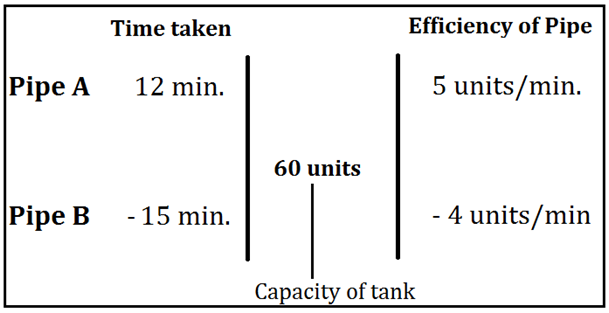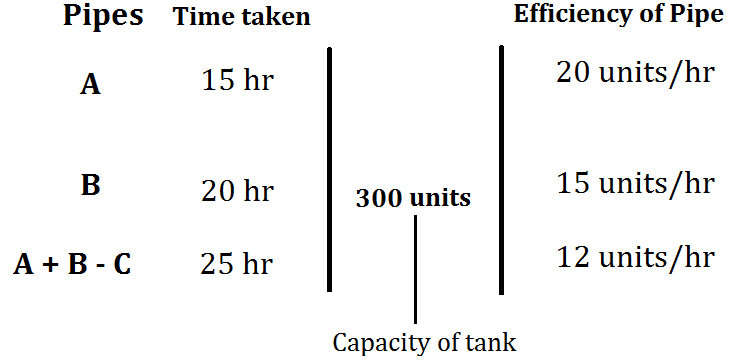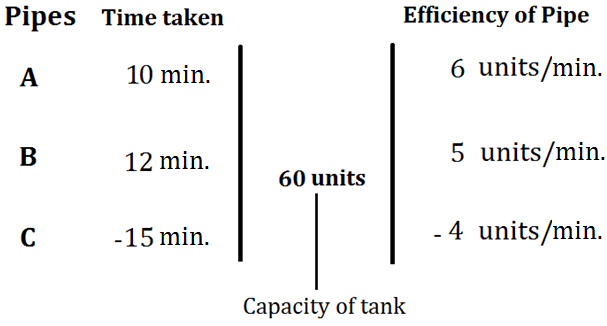# Pipes and Cistern Basic Concepts, Formulas, Tricks and Example Problems

‘Pipe and cistern’ is the subtopic of ‘Time and work,’ so you need to learn all the basics of ‘Time and work’ to excel in this topic. It involves the concept of negative work, so let us understand the basics of this topic and pattern of questions asked in different exams.

Cistern: Cistern is a tank for storing liquids generally water and has inlet and outlet pipes to fill or empty itself.

Pipe: Pipe is a section of a hollow cylinder for the discharge of water from one place to another. Pipes are generally connected to the cistern/tank for filling and emptying the cistern or tank.

Pipes are of two kinds:

Inlet Pipe: Pipe, which fills a cistern or tank, is an inlet pipe.

Outlet Pipe: Pipe, which empties a cistern or tank, is an outlet pipe.

Concept of Negative work

If a pipe A fills the tank and Pipe B empties the same tank, and we consider the work of filling the tank by pipe A as positive work, then work done by B is considered as negative work.

Important Points:

• If an inlet pipe fills a cistern or tank in x hours, then the part of the tank filled by the inlet tank in 1 hour will be $$1 \over x$$ times of the total capacity of the tank.
• If an outlet pipe empties a completely filled cistern or tank in y hours, then the part of tank emptied by outlet tank in 1 hour will be $$1 \over y$$ times of the total capacity of the tank.
• If both the inlet and outlet tank are opened together into a cistern, then part of the tank filled by both the pipes in 1 hour will be

$$=\dfrac{1}{x}-\dfrac{1}{y}$$

• If both the inlet and outlet tank are opened together into a cistern, then part of tank emptied by both the pipes in 1 hour will be

$$=\dfrac{1}{y}-\dfrac{1}{x}$$

• An outlet pipe can work if and only if the cistern or tank is already filled.

Let us understand the type of questions asked in different exams

Type 1: Pipe A can fill the tank in 12 minutes, and Pipe B can empty the tank in 15 minutes. If both the pipes are opened together, then how much time will be required to fill or empty the tank?

Solution: Let us assume the time to fill or empty the tank be X minutes

According to the question,

Pipe A can fill the tank in 12 minutes

Part of the tank filled by pipe A in 1 minute=$$1 \over 12$$

Pipe B can empty the tank in 15 minutes

Part of tank emptied by pipe B in 1 minute=$$1 \over 15$$

When both the pipes are opened together, part of the tank filled in one minute will be

$$⇒\dfrac{1}{12}-\dfrac{1}{15}=\dfrac{1}{60}$$

As there is a positive sign with 1/60 so we can say that tank will be filled and not emptied.

So, the time required to fill the tank will be = X = 60 minutes

Alternative: Let us assume the capacity of the tank be LCM (12, 15) = 60 units

Now, according to the questionWe know, the capacity of tank = time taken to fill tank * Efficiency of Pipe

So, from the above figure,

The efficiency of pipe A and pipe B together will be = 5 – 4 = 1 unit/min

Now, Time taken by pipe A and B together to fill the tank will be

$$⇒\dfrac{60}{1}=60 \space minutes$$

Type 2:  Pipe A and Pipe B alone can fill the tank in 15 hours and 20 hours, respectively. Pipe C is an outlet pipe. If all the pipes are opened together, then the tank gets entirely filled in 25 hours. In how many hours Pipe C can empty the half-filled tank?

Solution: Let us assume the time taken by pipe C to empty the completely filled tank be X hours

So, part of tank filled by pipe C in 1 hour will be=$$-1 \over X$$

Pipe A alone can fill the tank in 15 hours

Part of the tank filled by Pipe A in 1 hour=$$1 \over 15$$

Pipe B alone can fill the tank in 20 hours

Part of the tank filled by Pipe B in 1 hour=$$1 \over 20$$

Now, according to the question, if all the pipes are opened together, then the tank gets filled completely in 25 hours

$$⇒-\dfrac{1 }{ X} +\dfrac{1 }{ 15}+\dfrac{1 }{ 20}=\dfrac{1 }{25}$$

Solving the above equation for X we get,

$$⇒X=\dfrac{300 }{ 23}\space hours$$

Therefore, time taken by pipe C to empty the half-filled tank = X/2

$$⇒\dfrac{X}{2}=\dfrac{150 }{23}\space hours=6\dfrac{12}{23}\space hours$$

Alternative: Let us assume the capacity of the tank be LCM (15, 20 and 25) = 300 units

Now, according to the questionWe know, the capacity of tank = time taken to fill tank * Efficiency of Pipe

So, from the above figure,

The efficiency of pipe C will be = (Efficiency of A + Efficiency of B) – Efficiency of A + B – C

Efficiency of pipe C will be = 20 + 15 – 12 = 23 units/day

Now, Time taken by pipe C to empty the half-filled tank will be

$${⇒\dfrac{Capacity \space of \space tank}{Efficiency \space of \space C}=\dfrac{\dfrac{1}{2}*300}{23}=\dfrac{150}{23} \space hours=6\dfrac{12}{13} \space hours}$$

Type 3: Pipe A and Pipe B alone can fill the tank in 10 minutes and 12 minutes, respectively, while Pipe C can empty the tank in 15 minutes. If all the pipes are opened together, but after 4 minutes, pipe A and B are closed, then in how much time tank will get emptied again?

Solution: Let us assume the capacity of the tank be LCM (10, 12, and 15) = 60 units

Now, according to the questionWe know, the capacity of tank = time taken to fill tank * Efficiency of Pipe

So, from the above figure,

Efficiency of A, B and C together will be = 6 + 5 – 4 = 7

So, amount of tank filled by pipes A, B and C together in 5 minutes will be = 7 * 4 = 28 units

Now, volume of tank to be emptied by pipe C will be = 28 units

Time taken by Pipe C to empty 28 units of capacity of tank will be

$$⇒\dfrac{28}{4}=7 \space minutes$$

### BIGGEST SALE EVER get 35% Off Use Coupon code SUCCESS20240Current Affairs

Attempt Quiz

x